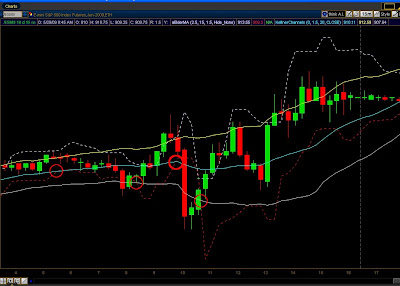## Friday, May 29, 2009

### Bull-Bear Magic plain and simple.Apply The Ketler Channel Study to you chart with this magic. If you're a Bear watch for the red lower dashed line to cross the KC midline for a sell(in some cases you must visually project the KC midline when price action is moving quickly), exit your short when the red dashed line crosses the KC lower band.

If you're a Bully do the opposite with the upper dashed line. Enjoy.input tolerance = 1.5;
input Length = 15;
input coeff = 2.5;
input hideLines = {Hide_Hi, Hide_Lo, Hide_Both, default Hide_None};

def h_hi;
def h_lo;
switch(hideLines){
case Hide_Hi:
h_hi = 1; h_lo = 0;
case Hide_Lo:
h_hi = 0; h_lo = 1;
case Hide_Both:
h_hi = 1; h_lo = 1;
default:
h_hi = 0; h_lo = 0;
}
def hi_Avg = sum(if(high > high,high - high,0), Length) / sum(if(high > high,1,0), Length);
def hi_line = high + (hi_Avg * coeff);
def hi_max = Min(Min(hi_line, hi_line), hi_line);
plot hi_plot = hi_max;
hi_plot.setStyle(Curve.Short_Dash);
hi_plot.setHiding(h_hi);

def lo_Avg = sum(if(low < low,low - low,0), Length) / sum(if(low < low,1,0), Length);
def lo_line = low - (lo_Avg * coeff);
def lo_max = Max(Max(lo_line, lo_line), lo_line);
plot lo_plot = lo_max;
lo_plot.setStyle(Curve.Short_Dash);
lo_plot.setHiding(h_lo);

plot magic = if( (hi_max-lo_max) <= tolerance, hi_max,double.nan);
magic.setPaintingStrategy(PaintingStrategy.POINTS);